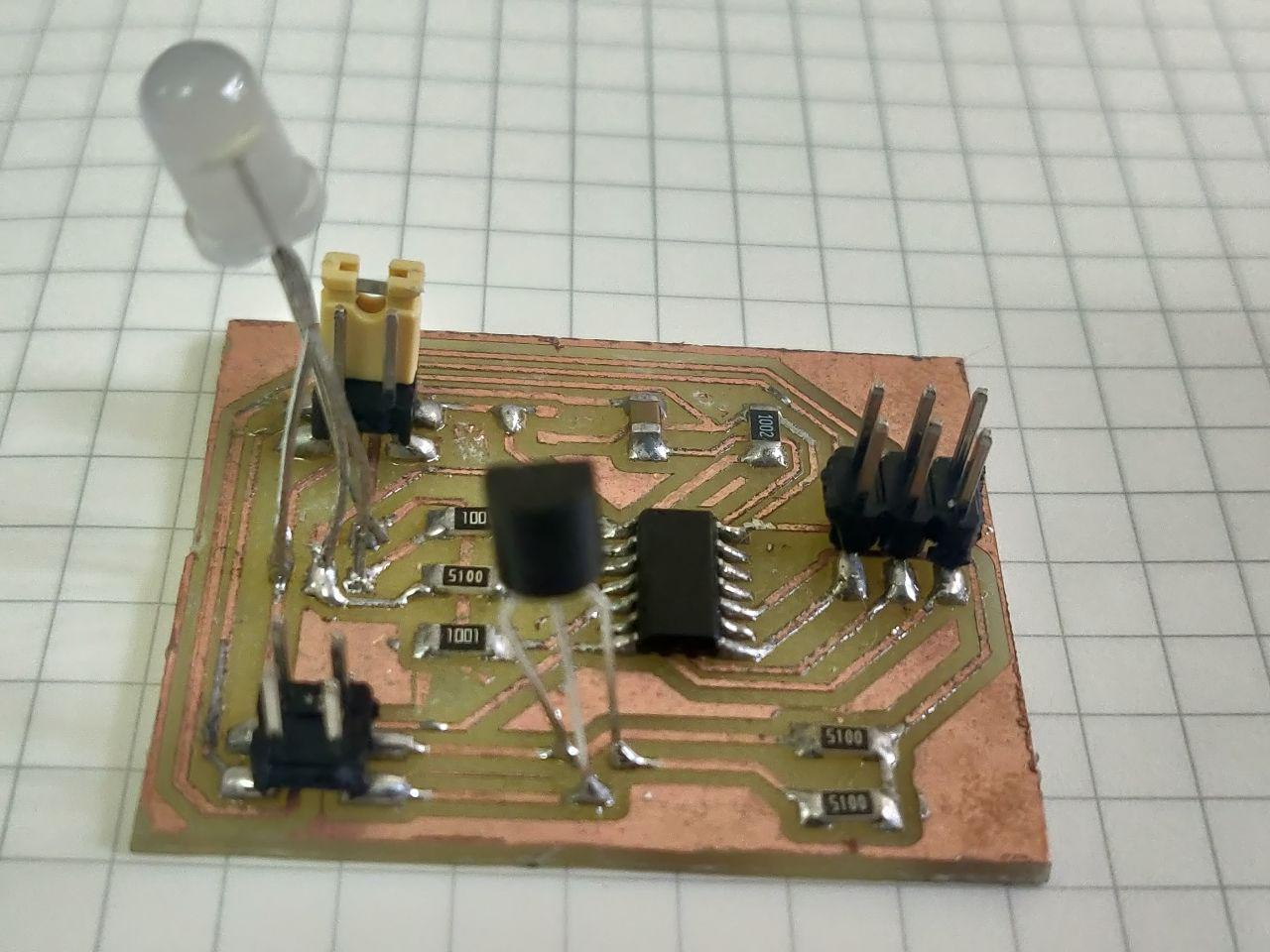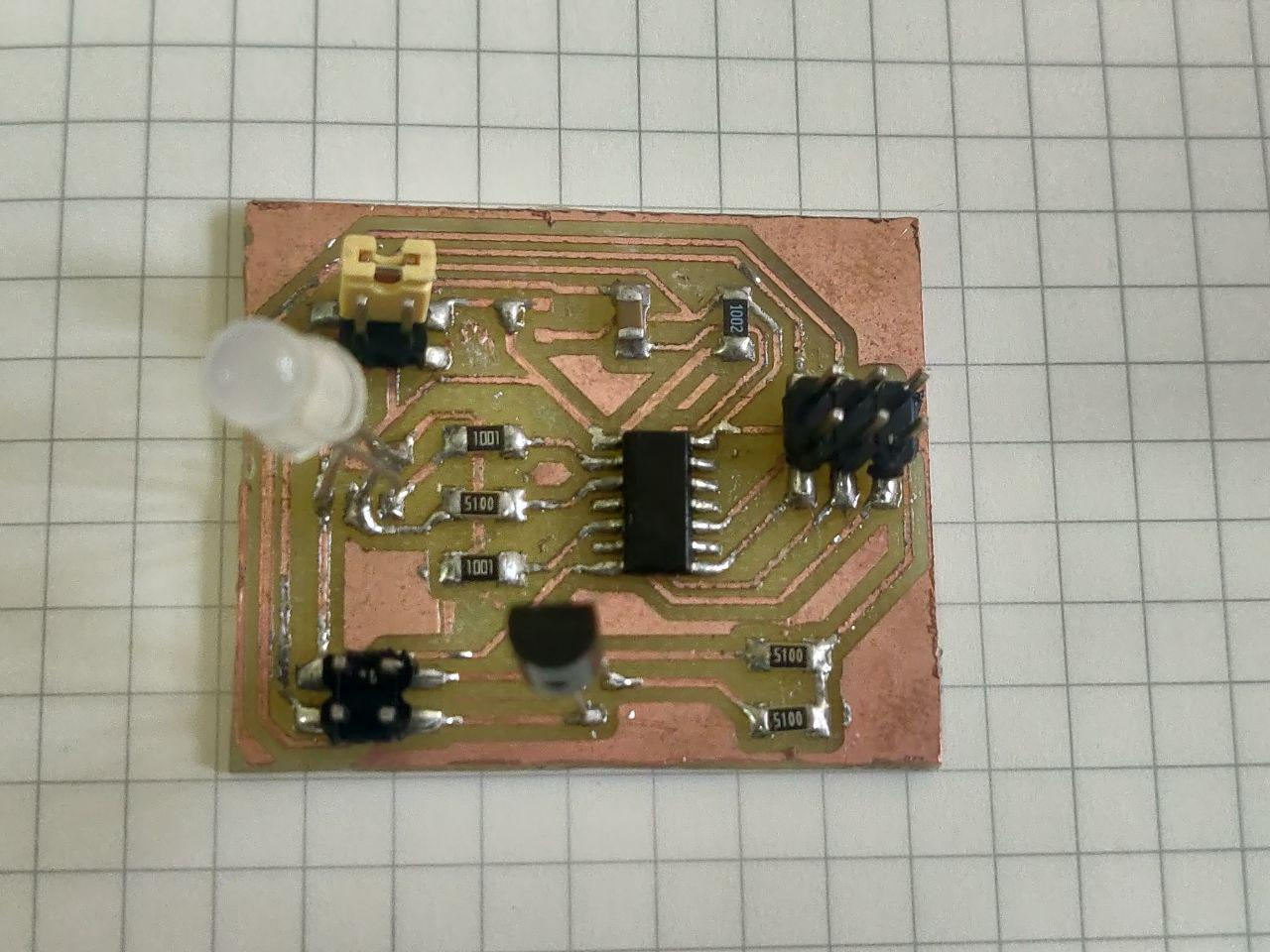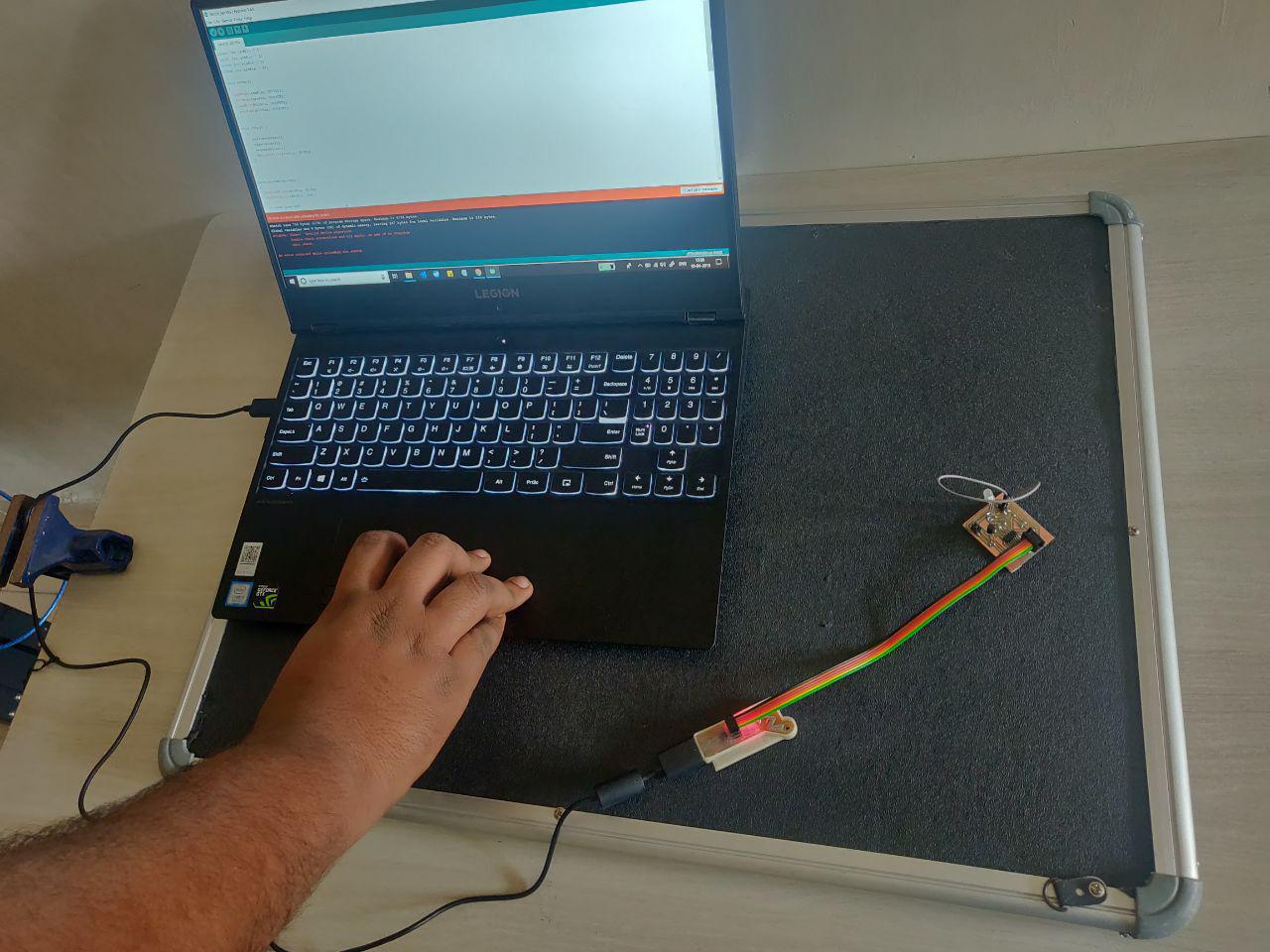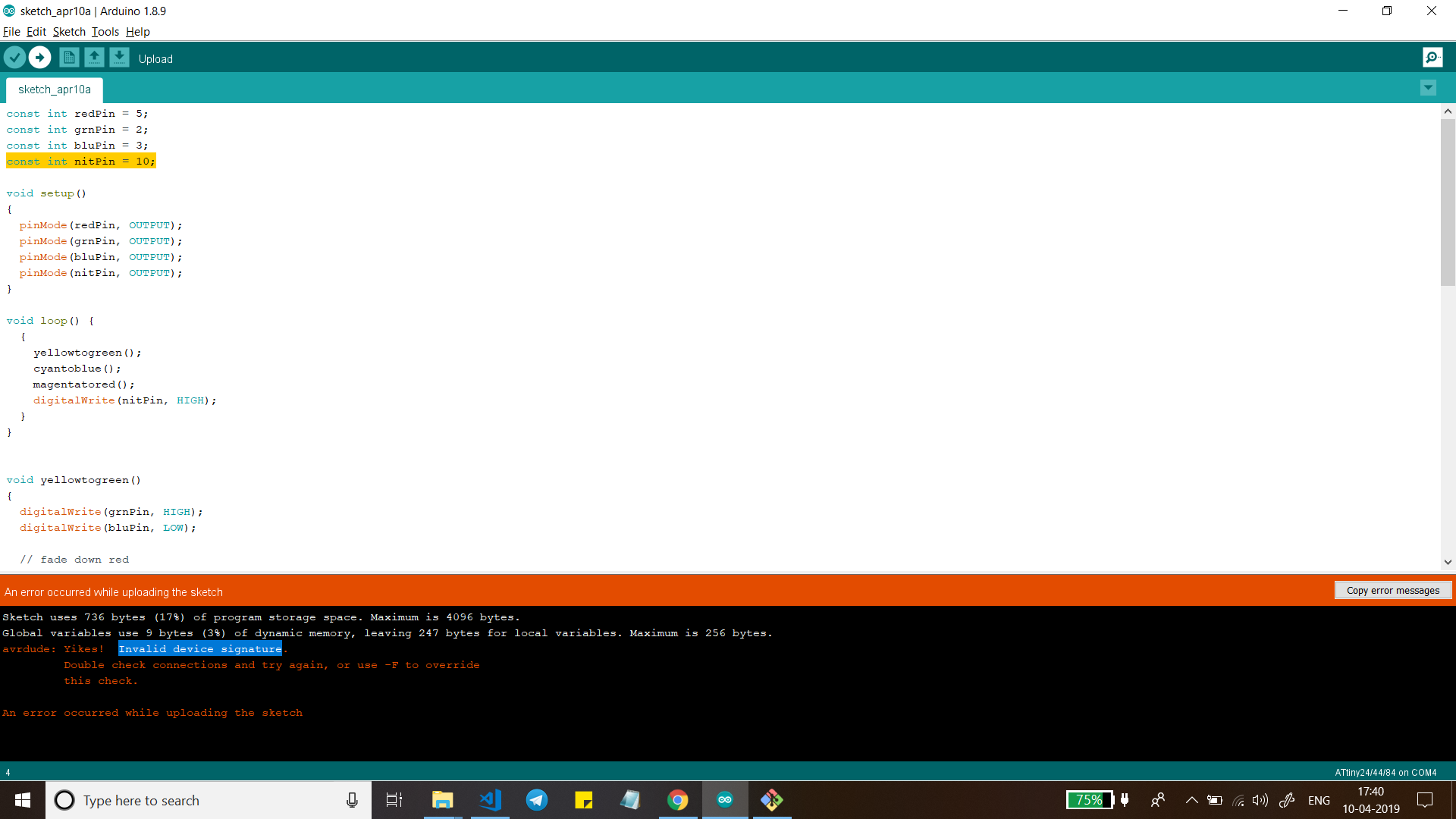# 12. Output devices¶

This week the assignments are:

• Individual assignment: Add an output device to a microcontroller board you’ve designed, and program it to do something

• Group assignment: Measure the power consumption of an output device

## Board design¶

This week I designed my board to have two different outputs one is an RGB LED and the other is for a shape memory alloy called Nitinol.

Since there are two different outputs I thought of using ATTiny44 since it has more interrupts(IO pins). I could have used ATTiny45 but since I might require the extra pins I didn’t use it.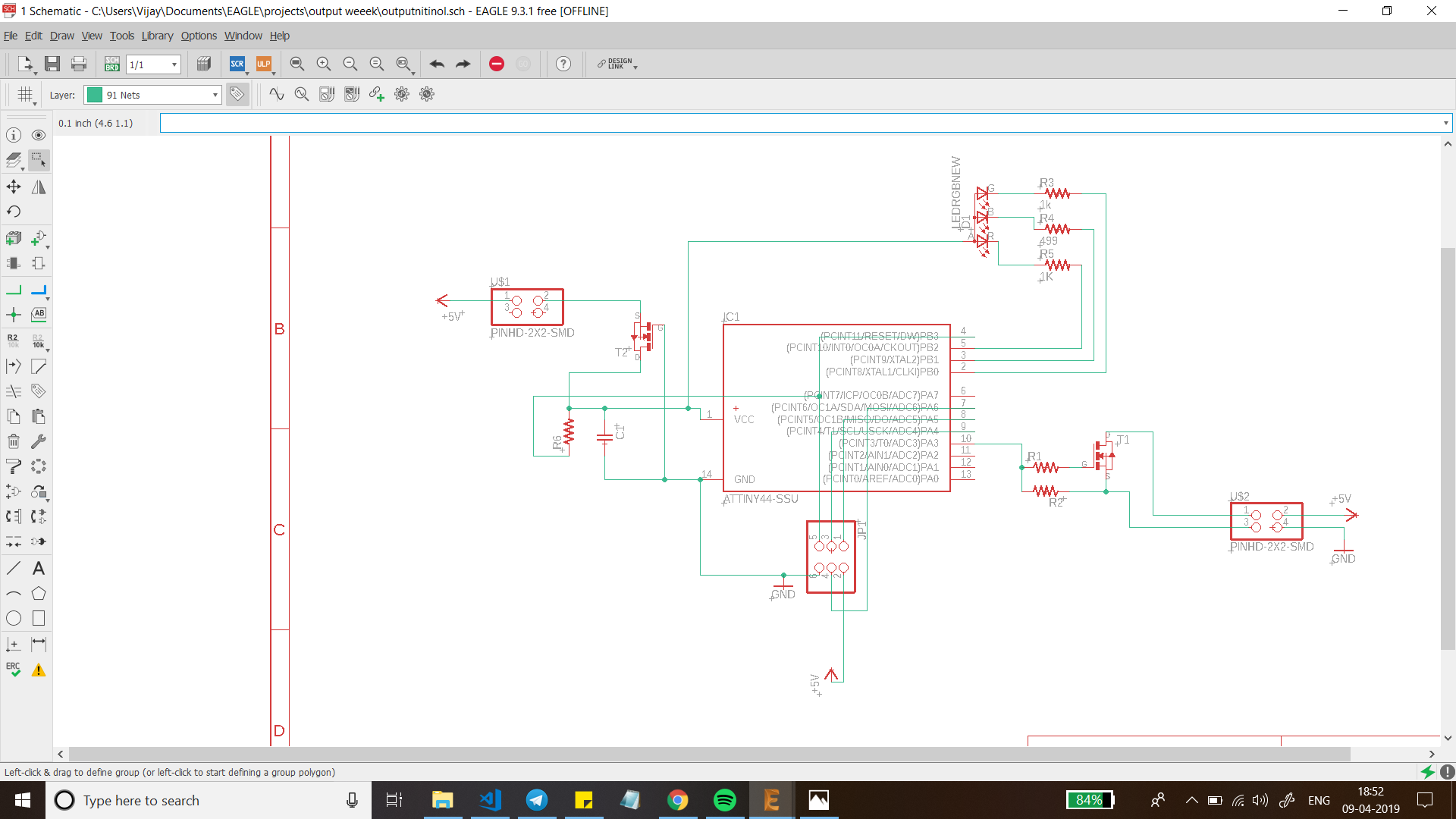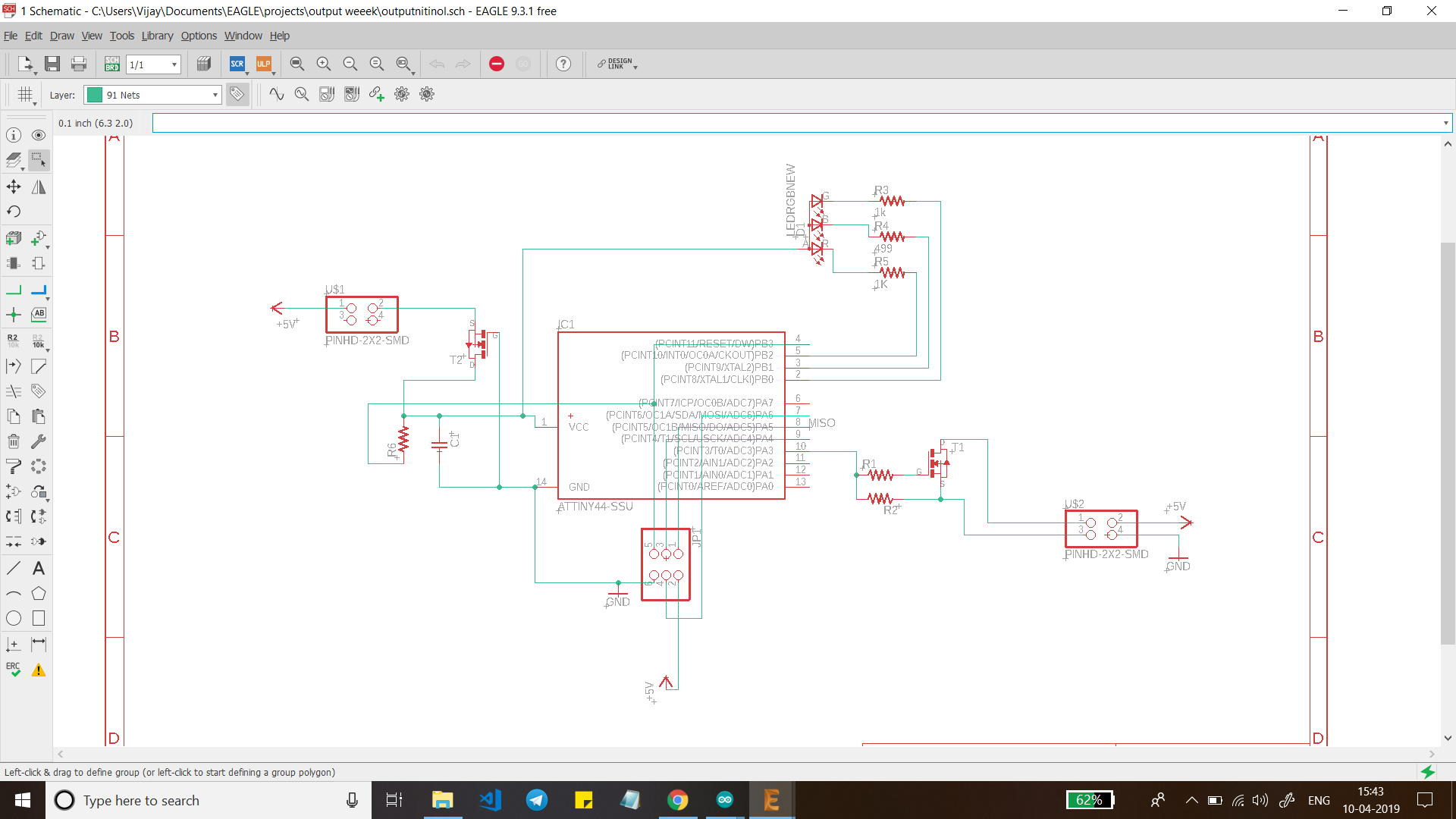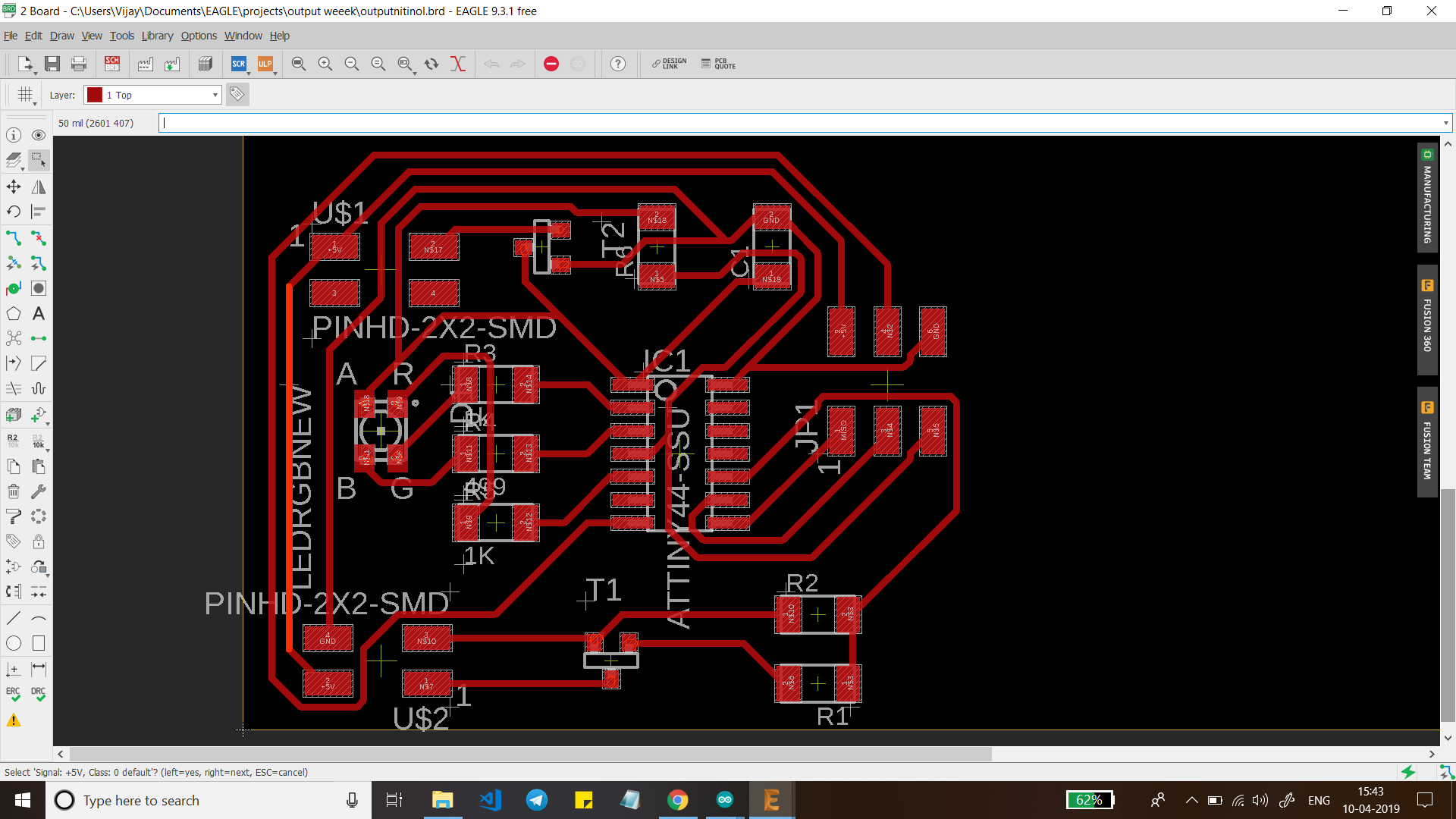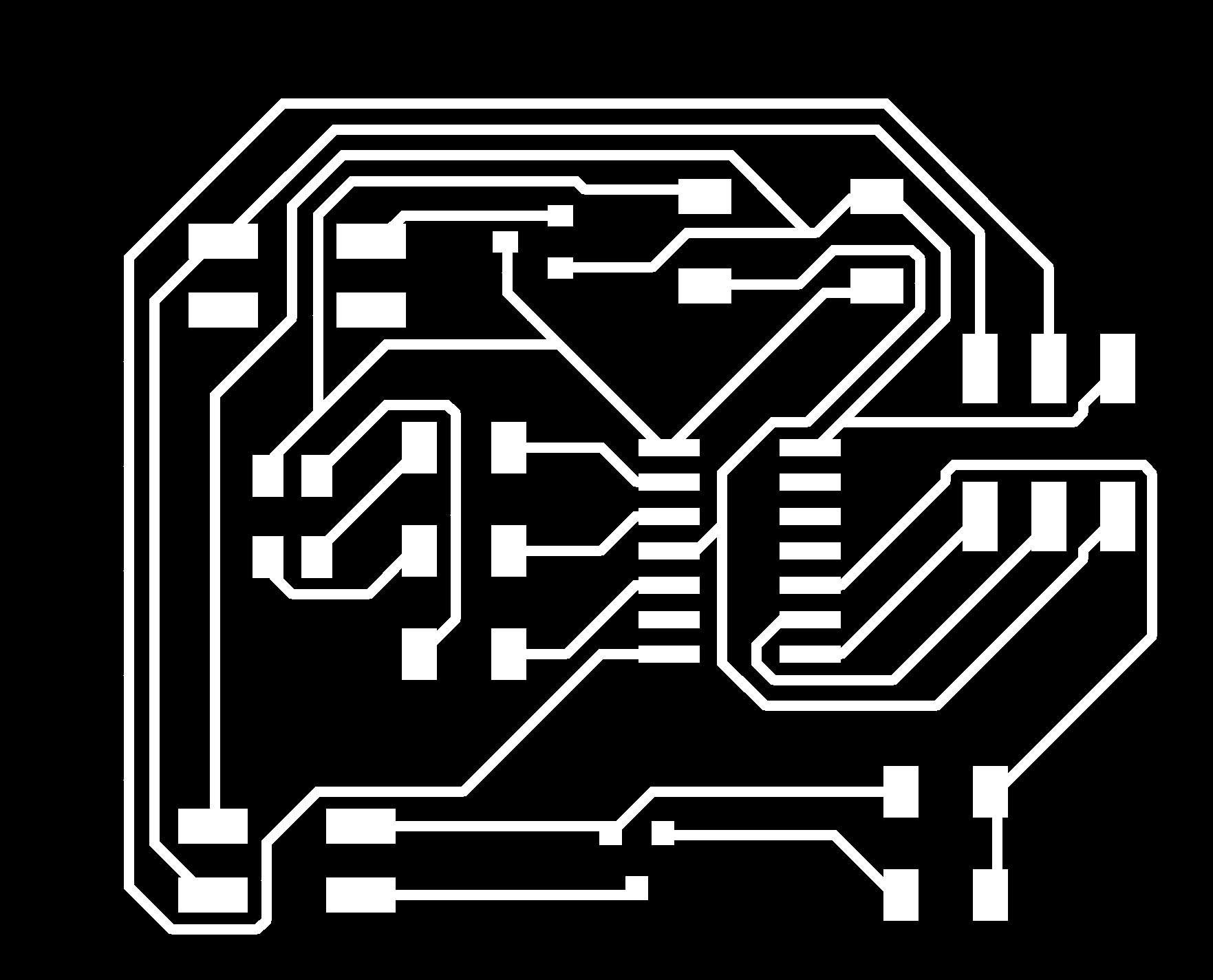### Nitinol¶

This portion of the circuit is similar to the Flapping Origami Crane Project from the archive but there is a key difference, I am using only one long Nitinol wire.

This is just for demonstration nothing more.

When designing a circuit aroung Nitinol We have to consider its mechanical properties and we have to follow the limitations set by the manufacturer the reason being that it is an Expensive material and exceeding the current limits will burn the wire. The data sheet of a Nitinol wire gives us the following information: * Diameter * Resistance * Current rating * Reshaping temperature

While designing the circuit resistance and current rating are important. DO NOT exceed the limits.

Nitinol works by contracting when it is heated up, in order to do that we have to pass current trough the wire since it has high resistance it gets hot and contracts. If we let the current pass for too long the wire gets hot and burns up.

Think of it as a cross between nichrome wire and solder wire.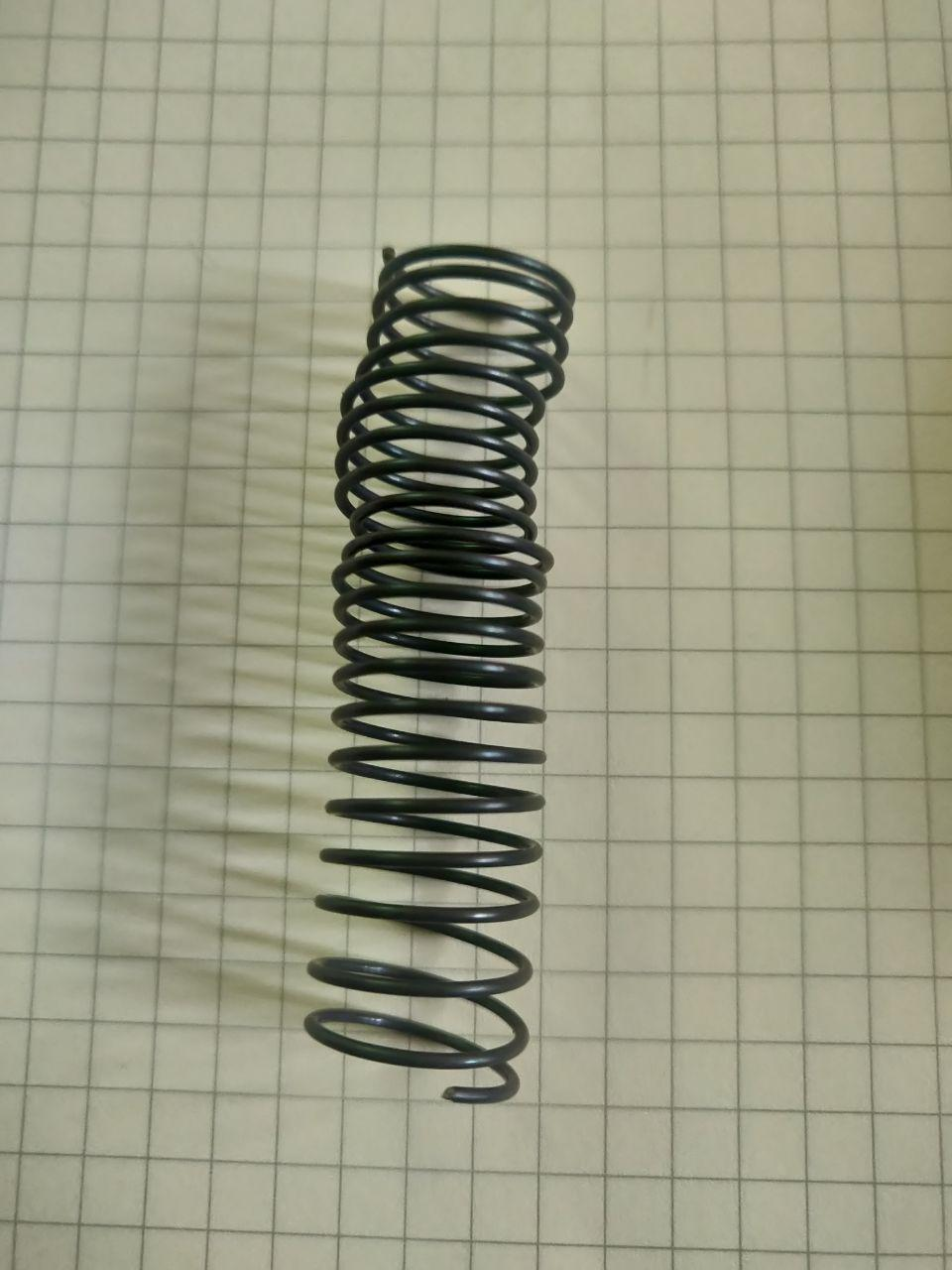### RGB LED¶

This part of the circuit is kind of similar to the circuit from the Archive, after looking at the ciruit it was a simple circuit. I am using a common anode RGB LED. The LED has four pins where one pin is the anode and the other three pins are assigned to each of the primary color(R,G,B) and each color of R,G,B is assigned a resistor.The purpose of putting this portion(Even though it is unneccessary…) of the circuit was to experiment with multiple outputs since I will have multiple outputs in my final project.

## Code Example¶

The Code is a two part code for the RGB LED which was taken from the archive.

the code below is only for RGB LED

```#include <avr/io.h>
#include <util/delay.h>

#define output(directions,pin) (directions |= pin) // set port direction for output
#define set(port,pin) (port |= pin) // set port pin
#define clear(port,pin) (port &= (~pin)) // clear port pin
#define pin_test(pins,pin) (pins & pin) // test for port pin
#define bit_test(byte,bit) (byte & (1 << bit)) // test for bit set
#define PWM_delay() _delay_us(25) // PWM delay

#define led_port PORTB
#define led_direction DDRB
#define red (1 << PB1)
#define green (1 << PB0)
#define blue (1 << PB2)

int main(void)
{

unsigned char count, pwm;

CLKPR = (1 << CLKPCE);
CLKPR = (0 << CLKPS3) | (0 << CLKPS2) | (0 << CLKPS1) | (0 << CLKPS0);

set(led_port, red);
output(led_direction, red);
set(led_port, green);
output(led_direction, green);
set(led_port, blue);
output(led_direction, blue);

while (1)
{

for (count = 0; count < 255; ++count)
{
clear(led_port,red);
for (pwm = count; pwm < 255; ++pwm)
PWM_delay();
set(led_port,red);
for (pwm = 0; pwm < count; ++pwm)
PWM_delay();
}

for (count = 0; count < 255; ++count)
{
set(led_port,red);
clear(led_port,green);
for (pwm = count; pwm < 255; ++pwm)
PWM_delay();
clear(led_port,red);
set(led_port,green);
for (pwm = 0; pwm < count; ++pwm)
PWM_delay();
}

for (count = 0; count < 255; ++count)
{
set(led_port,green);
clear(led_port,blue);
for (pwm = count; pwm < 255; ++pwm)
PWM_delay();
clear(led_port,green);
set(led_port,blue);
for (pwm = 0; pwm < count; ++pwm)
PWM_delay();
}

for (count = 0; count < 255; ++count)
{
set(led_port,blue);
clear(led_port,green);
clear(led_port,red);
for (pwm = count; pwm < 255; ++pwm)
PWM_delay();
set(led_port,blue);
set(led_port,green);
set(led_port,red);
for (pwm = 0; pwm < count; ++pwm)
PWM_delay();
}
for (count = 0; count < 255; ++count)
{
set(led_port,blue);
set(led_port,green);
set(led_port,red);
for (pwm = count; pwm < 255; ++pwm)
PWM_delay();
clear(led_port,blue);
clear(led_port,green);
clear(led_port,red);
for (pwm = 0; pwm < count; ++pwm)
PWM_delay();
}
}
}
```

The next code is for both nitinol wire and RGB LED.

```const int redPin = 5;
const int grnPin = 2;
const int bluPin = 3;
const int nitPin = 10;

void setup()
{
pinMode(redPin, OUTPUT);
pinMode(grnPin, OUTPUT);
pinMode(bluPin, OUTPUT);
pinMode(nitPin, OUTPUT);
}

void loop() {
{
yellowtogreen();
cyantoblue();
magentatored();
digitalWrite(nitPin, HIGH);
}
}

void yellowtogreen()
{
digitalWrite(grnPin, HIGH);
digitalWrite(bluPin, LOW);

for(byte i=1; i<100; i++) {
byte on  = 100-i;
byte off = i;
for( byte a=0; a<100; a++ ) {
digitalWrite(redPin, HIGH);
delayMicroseconds(on);
digitalWrite(redPin, LOW);
delayMicroseconds(off);
}
}
}

void cyantoblue()
{
digitalWrite(bluPin, HIGH);
digitalWrite(redPin, LOW);

for(byte i=1; i<100; i++) {
byte on  = 100-i;
byte off = i;
for( byte a=0; a<100; a++ ) {
digitalWrite(grnPin, HIGH);
delayMicroseconds(on);
digitalWrite(grnPin, LOW);
delayMicroseconds(off);
}
}
}

void magentatored()
{
digitalWrite(redPin, HIGH);
digitalWrite(grnPin, LOW);

for(byte i=1; i<100; i++) {
byte on  = 100-i;
byte off = i;
for( byte a=0; a<100; a++ ) {
digitalWrite(bluPin, HIGH);
delayMicroseconds(on);
digitalWrite(bluPin, LOW);
delayMicroseconds(off);
}
}
}
```

## Results¶

Unfortunately this week’s board ended as failure. There were quite a few mistakes, be it the components selection or the board. I made a few mistakes while designing the board.

Firstly, I didn’t check the inventory to see wether the lab had common anode RGB LED.
Secondly, I didn’t utilze the the two pin headers properly and lastly, silly mistakes here and there.

I had several errors which were Identifiable bythis helpful pdf file by a Student